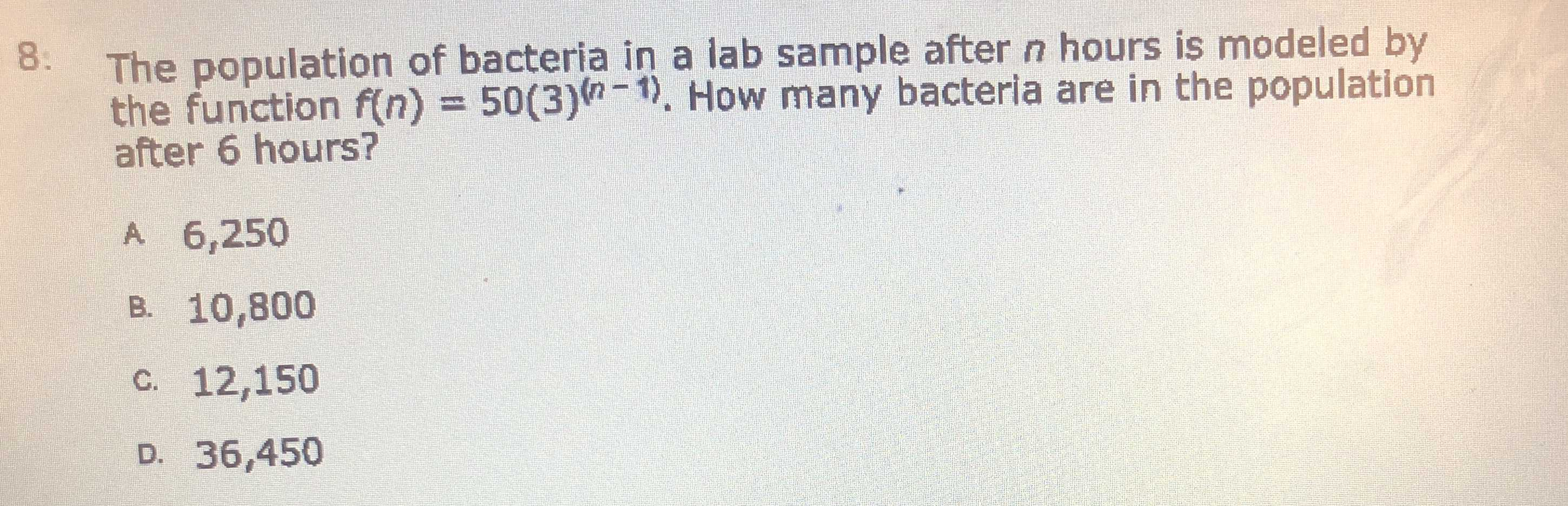### ¿Todavía tienes preguntas de matemáticas?

Pregunte a nuestros tutores expertos
Algebra
Pregunta8: The population of bacteria in a lab sample after $$n$$ hours is modeled by the function $$f ( n ) = 50 ( 3 ) ^ { ( n - 1 ) }$$ . How many bacteria are in the population after $$6$$ hours?

A. $$6,250$$

B. $$10,800$$

C. $$12,150$$

D. $$36,450$$